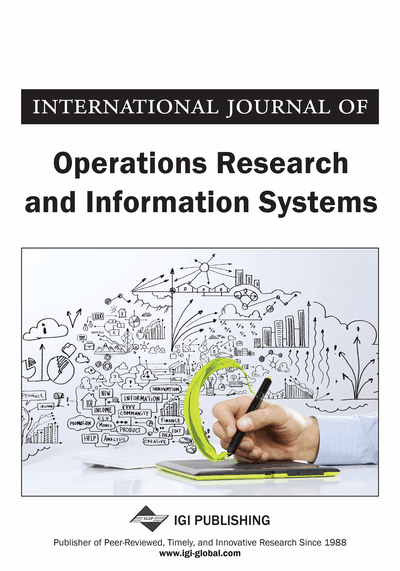# Random Node Pair Sampling-Based Estimation of Average Path Lengths in Networks

Luis E. Castro (Department of Industrial Engineering, University of Miami, Coral Gables, USA) and Nazrul I. Shaikh (Department of Industrial Engineering, University of Miami, Coral Gables, USA)
DOI: 10.4018/IJORIS.2018070102
Available
\$33.75
List Price: \$37.50
10% Discount:-\$3.75
TOTAL SAVINGS: \$3.75

## Abstract

This article describes how the average path length (APL) of a network is an important metric that provides insights on the interconnectivity in a network and how much time and effort would be required for search and navigation on that network. However, the estimation of APL is time-consuming as its computational complexity scales nonlinearly with the network size. In this article, the authors develop a computationally efficient random node pair sampling algorithm that enables the estimation of APL with a specified precision and confidence. The proposed sampling algorithms provide a speed-up factor ranging from 240-750 for networks with more than 100,000 nodes. The authors also find that the computational time required for estimation APL does not necessarily increase with the network size; it shows an inverted U shape instead.
Article Preview
Top

## 1. Introduction

The average of the shortest paths between any two nodes of a network is a global metric of high relevance. Popularly called as the average path length (APL), it provides useful insights on the level of interconnectivity in a network and the time it would take for information/goods to flow between any two randomly selected points on the network. APL has been shown to be an important metric for tasks such as the designing of real-life transportation networks (Balmer et al., 2004; Klunder and Post, 2006; Ziliaskopoulos et al., 1997), design of routing networks (Costa et al., 2007; Dabek et al., 2004), design of web-based networks (Backstrom et al., 2012; Fu et al., 2008; Kleinberg, 2000; Newman, 2000), studying propagation of diseases (Dekker, 2013), diffusion of information and opinion dynamics (Yildiz et al., 2011). Researchers have also shown that search and navigation are easier when APL is small (Zhang et al., 2008). However, estimating APL takes a lot of time and is sometimes infeasible on account of lack of computational resources (Wang, 2006).

Researchers have shown that he computational time required to estimate APL scale aswhereis the number of vertices anda function of the number of edges in the network (Madduri et al., 2007).scales aswhere. This quadratic to cubic scaling of the computational time with network size makes the estimation of APL impractical as the network sizes increase (Wang, 2006). As an example, it takes approximately 9.6 hours to estimate the APL of a synthetic network created using the preferential attachment algorithm with about 100,000 nodes on a 16GB RAM PC and the time requirement increases to more than five days for a network with 1 Million nodes. Such a long wait time is generally impractical, especially in simulation and emulation studies that require generation of 100s of synthetic networks and the estimation of APL for each scenario.

In this paper, we develop a random node pair sampling based strategy to estimate the APL for mid-sized networks when both computational time and capacity are limited. Though sampling introduces some uncertainty in the reliability of the parameter estimates, the precision and confidence can be controlled using a combination of the right sampling strategy and sample size. We therefore propose and demonstrate the efficacy (regarding computational time, confidence level, and precision) of the proposed node pair sampling algorithm. We compare the proposed algorithm with random node sampling algorithm and algorithms wherein the node sampling is non-uniform. The random node pair sampling algorithm yields a speed up factor of more than 411 when compared to the algorithm that uses random node sampling and speed up of 750 when compared to the algorithm that measures APL using the population information. The proposed algorithm uses the central limit theorem approximation to determine the sample size for a given precision and confidence level.

## Complete Article List

Search this Journal:
Reset
Volume 14: 1 Issue (2023): Forthcoming, Available for Pre-Order
Volume 13: 2 Issues (2022)
Volume 12: 4 Issues (2021)
Volume 11: 4 Issues (2020)
Volume 10: 4 Issues (2019)
Volume 9: 4 Issues (2018)
Volume 8: 4 Issues (2017)
Volume 7: 4 Issues (2016)
Volume 6: 4 Issues (2015)
Volume 5: 4 Issues (2014)
Volume 4: 4 Issues (2013)
Volume 3: 4 Issues (2012)
Volume 2: 4 Issues (2011)
Volume 1: 4 Issues (2010)
View Complete Journal Contents Listing